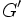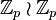# Characteristicity does not satisfy lower central series condition

This article gives the statement, and possibly proof, of a subgroup property (i.e., characteristic subgroup) not satisfying a subgroup metaproperty (i.e., lower central series condition).
View all subgroup metaproperty dissatisfactions | View all subgroup metaproperty satisfactions|Get help on looking up metaproperty (dis)satisfactions for subgroup properties
Get more facts about characteristic subgroup|Get more facts about lower central series condition|

## Statement

It is possible to have a group$G$ and a characteristic subgroup$H$ of$G$ such that there is a positive integer$k$ for which the lower central series member$\gamma_k(H)$ is not a characteristic subgroup of the lower central series member$\gamma_k(G)$.

In fact, we can construct, for each positive integer$k > 1$, an example that works for that$k$. In the special case that$k = 2$, we obtain an example of a group$G$ and a characteristic subgroup$H$ of$G$ such that the derived subgroup$H'$ is not a characteristic subgroup of the derived subgroup$G'$.

## Proof

### Example for the derived subgroup ($k = 2$)

#### Smallest order example for a 2-group

Further information: faithful semidirect product of E8 and Z4

Suppose$G$ is the faithful semidirect product of E8 and Z4. We can think of it as the semidirect product of elementary abelian group:E8 (a three-dimensional vector space over field:F2) and cyclic group:Z4, where the generator of the latter acts as the following matrix on the former:$\begin{pmatrix} 1 & 1 & 0 \\ 0 & 1 & 1 \\ 0 & 0 & 1 \\\end{pmatrix}$

•$\gamma_2(G) = G'$, the derived subgroup of$G$, is a Klein four-group inside the base of the semidirect product, generated by the first two basis vectors.
• Let$H = C_G(G')$.$H$ contains the base of the semidirect product as well as the square of the generator of cyclic group:Z4. Explicitly,$H$ is the semidirect product of the base by the element acting as follows:$\begin{pmatrix} 1 & 0 & 1 \\ 0 & 1 & 0 \\ 0 & 0 & 1 \\\end{pmatrix}$

•$H$ is isomorphic to direct product of D8 and Z2. The derived subgroup$\gamma_2(H) = H'$ of$H$ is isomorphic to cyclic group:Z2 (generated by the second basis vector) and it lives inside$G'$ as Z2 in V4. Thus,$H'$ is not a characteristic subgroup of$G'$.

#### Smallest order example for a 3-group

Further information: wreath product of Z3 and Z3

Let$G$ be the group$\mathbb{Z}_3 \wr \mathbb{Z}_3$. Explicitly,$G$ is the semidirect product of elementary abelian group:E27, viewed as a three-dimensional vector space over field:F3, with the acting element of order three acting by the matrix:$\begin{pmatrix} 0 & 0 & 1 \\ 1 & 0 & 0 \\ 0 & 1 & 0 \\\end{pmatrix}$

•$\gamma_2(G) = G'$ is the subgroup in the base comprising the elements whose coordinates add up to zero. It is an elementary abelian group of prime-square order.
•$H$ is defined as the internal semidirect product of$G'$ and any element of three outside the base. There is a unique possibility for such a subgroup$H$, and it is isomorphic to unitriangular matrix group:UT(3,3).
•$\gamma_2(H) = H'$ is a subgroup of order 3. It lives as a group of order 3 inside$G'$ which is elementary abelian of order 9, hence is not characteristic in$G'$.

### Example for larger$k$

Further information: wreath product of groups of prime order

For a positive integer$k$, choose$p$ to be a prime number greater than$k$.

• Define$G$ as the wreath product of groups of prime order$\mathbb{Z}_p \wr \mathbb{Z}_p$. This is a group of order$p^{p+1}$ with an elementary abelian normal subgroup of order$p^p$ and a complement of order$p$ acting by cyclic permutation of the coordinates.
• Define$H$ as the internal semidirect product of$G'$ with an element of order$p$ outside the elementary abelian normal subgroup.
•$\gamma_k(H)$ and$\gamma_k(G)$ are both elementary abelian subgroups of$G$, with$\gamma_k(H)$ having order$p^{p-k}$ and$\gamma_k(G)$ having order$p^{p-k+1}$. In particular,$\gamma_k(H)$ is a proper nontrivial subgroup of the elementary abelian$p$-group$\gamma_k(G)$, hence it is not characteristic inside$\gamma_k(G)$.